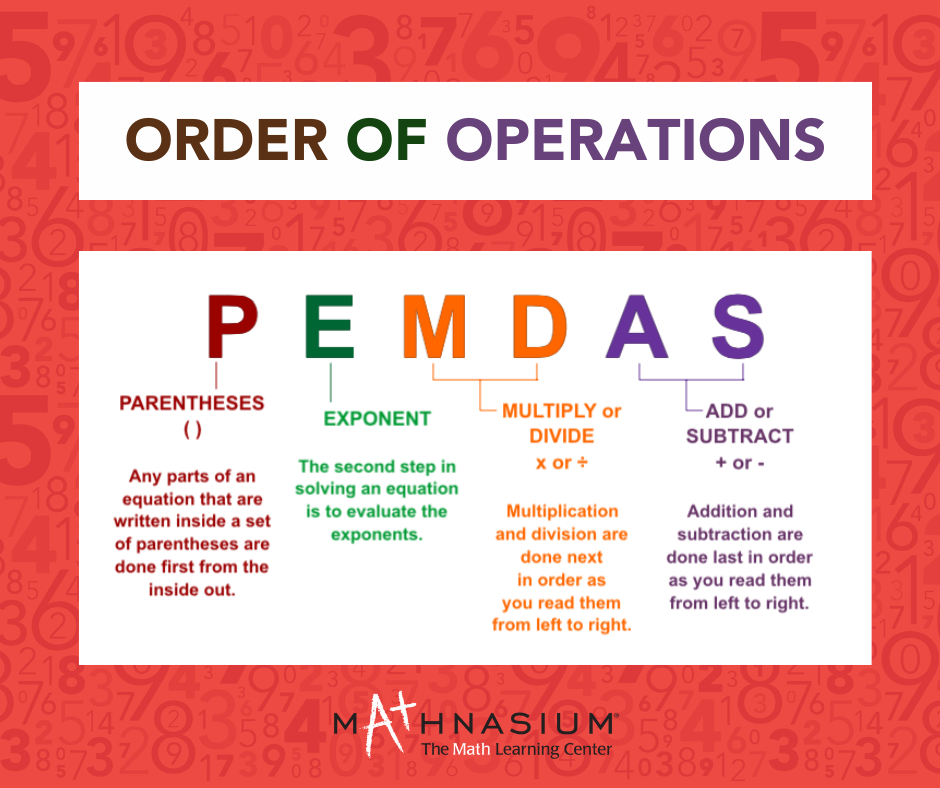877-601-6284
Get Started by Finding a Local Center

# What is PEMDAS?

Sep 30, 2022 | EaganMathematics is all around us, and the right mathematical rules can help you solve problems. The PEMDAS rule is one such rule in the mathematical domain. Math basics such as addition, division, subtraction, and multiplication are already familiar to you, but what about the right order to address each of them, were they all in the same equation? Did you know there was an order to solve them? PEMDAS is widely used in applications in mathematics, and computer programming and we use it to solve a wide range of problems.

What is PEMDAS?

PEMDAS is an acronym used to mention the order of operations to be followed while solving expressions having multiple operations. PEMDAS stands for P- Parentheses, E- Exponents, M- Multiplication, D- Division, A- Addition, and S- Subtraction. There are different acronyms used for the order of operations in different countries. For example, in Canada, the order of operations is stated as BEDMAS (Brackets, Exponents, Division, Multiplication, Addition, and Subtraction). Some people prefer to say BODMAS (B- Brackets, O- Order or Off), while few others call it GEMDAS (G- Grouping).

John's Method
5+2×3
= 7×3
= 21

Bob's Method

5+2×3
=5+6
=11

As you can observe, John and Bob reached different answers. There can only be one correct answer to this expression in mathematics! Can you decide who is correct?

PEMDAS Rules

These rules start with Parentheses, and then operations are performed on the exponents or powers. Next, we perform operations on multiplication or division from left to right. Finally, operations on addition or subtraction are performed from left to right. You will always get the correct answer if you follow the PEMDAS rule.

Let us understand PEMDAS with the help of an example.

4+3[8-2(6-3)]÷2

We will begin with working from the inside of the brackets. We will solve the innermost bracket first and then move outside.

• Starting with 6 – 3 = 3, we get: 4 + 3[8 – 2(3)] ÷ 2
• Next, multiplying 2(3)=6 or 2×3=6, we get: 4 + 3[8 – 6] ÷ 2
• There is one bracket left, [8 – 6] = 2, we get: 4 + 3 ÷ 2
• Solving 3 or 3 × 2 = 6, we have: 4 + 6 ÷ 2

We can observe that all the expressions in the brackets are solved. Based on PEMDAS, we know that division comes next, hence, 6 ÷ 2 = 3, that is, 4 + 3. And lastly, addition 4 + 3 = 7.

Use this rule to solve math questions and soar in your math class.

Till then, keep practicing!

##### Locations near
Could not find Center, try again